﻿ 粮食产量影响因素的多元线性回归分析

# 粮食产量影响因素的多元线性回归分析Multiple Linear Regression Analysis of Factors Affecting Grain Yield

Abstract: This paper mainly introduces how to use multivariate linear regression method to analyze the in-fluencing factors of grain yield, and use IBM SPSS Statistics software to carry out analysis, and put forward relevant suggestions based on the analysis results.

1. 引言

2018年中共中央、国务院印发了《乡村振兴战略规划(2018-2022年)》，其中要求贯彻落实加快农业现代化步伐，提升农业科技创新水平。农业也是国家发展的基础  ，粮食产量更是我国经济发展不可缺少的指标。相关资料显示，国家近几年来的粮食产量在不断提高，而粮食产量与哪些因素有关，关系如何等问题便成了要进一步提高粮食产量必须要解决的问题。

2. 多元线性回归相关理论

2.1. 多元线性回归方程

$f\left({x}_{t}\right)={a}_{0}+{a}_{1}{x}_{1t}+\cdots +{a}_{n}{x}_{nt}+{\mu }_{t}$

1) 自变量对因变量有显著的影响，并呈现出非确定关系(线性关系)；

2) 样本容量 > 回归系数的个数；

3) 残差服从正态分布。

2.2. 回归系数估计(OLS)

$Q\left({\beta }_{0},{\beta }_{1},\cdots ,{\beta }_{k}\right)=\sum \left({y}_{t}-{\beta }_{0}-{\beta }_{1}{x}_{1t}-\cdots -{\beta }_{n}{x}_{nt}\right)$

$n{\beta }_{0}+{\beta }_{1}\sum {x}_{1t}+\cdots +{\beta }_{n}\sum {x}_{nt}=\sum {y}_{t}$

${\beta }_{0}\sum {x}_{1t}+{\beta }_{1}\sum {x}_{1t}{}^{2}+\cdots +{\beta }_{n}\sum {x}_{1t}{x}_{nt}=\sum {x}_{1t}{y}_{t}$

$\cdots \text{ }\text{ }\text{ }\text{ }\text{ }\text{ }\text{ }\text{ }\text{ }\text{ }\text{ }\cdots$

${\beta }_{0}\sum {x}_{ni}+{\beta }_{1}\sum {x}_{1t}{x}_{nt}+\cdots +{\beta }_{n}\sum {x}_{nt}{}^{2}=\sum {x}_{nt}{y}_{t}$

2.3. 总方差估计及显著性检验

${S}^{2}=\frac{\sum {e}_{t}{}^{2}}{m-n}$

3. 根据数据构建回归方程

3.1. 构建回归方程Table 1. Food production data for 2008-2017

1) 如表2所示，自变量对因变量有显著的影响，并呈现出非确定关系(线性关系)；

2) 10 (样本容量) > 6(系数个数)；

3) 如图1所示，残差服从正态分布。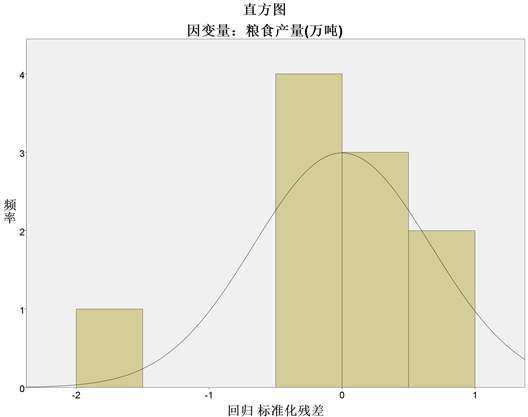Figure 1. Standardized residual diagram

$f\left(x\right)={\beta }_{0}+{\beta }_{1}{x}_{1}+{\beta }_{2}{x}_{2}+{\beta }_{3}{x}_{3}+{\beta }_{4}{x}_{4}+{\beta }_{5}{x}_{5}+\mu$

3.2. 求解回归方程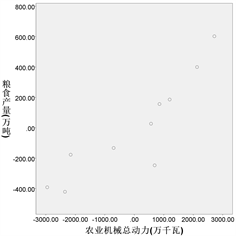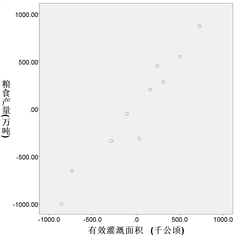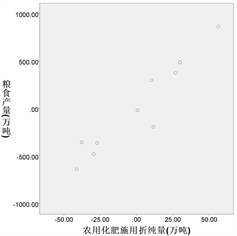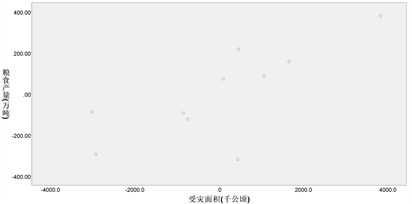Figure 2. Partial regression diagrams of dependent and independent variables

$f\left(x\right)=114085.247-2.018{x}_{1}+0.155{x}_{2}+1.099{x}_{3}+14.134{x}_{4}-0.081{x}_{5}$

3.3. 显著性检验Table 3. Test indicators and data values

4. 结合实际给出相关建议

1) 通过线性回归方程可以看出，化肥施用量与粮食产量的正相关性最强，所以化肥可以有效促进粮食产量的提高。但在施用化肥的过程中要适量，否则会污染环境、降低土地肥力等，不利于长期发展。

2) 有效灌溉面积与粮食产量相关性较强，所以在粮食生产过程中，应有适量的水分，即在雨水不充足时采用人工降雨等措施。

3) 根据数据显示，播种面积与粮食产量成负相关，主要原因为生产技术的提高使得，即使是减小播种面积也可以增加粮食的产量。即生产力的发展对粮食产量的提高有重大作用，可节约农业用地，使土地得到更合理的利用。所以应该合理的规划已有用地，提高生产质量，进而提高粮食产量。

4) 成灾面积与粮食产量成负相关，即应尽量减少成灾面积，减少粮食产量的损失。如及时给农作物驱赶害虫，洪涝灾害时及时排水等。

 李妍. 中国粮食生产因素及地区差异分析[J]. 经济研究导刊, 2009(13): 45-46.

 向东进, 李宏伟, 刘小雅. 实用多元统计分析[M]. 武汉: 中国地质大学(武汉)出版社, 2005.

 单良, 胡勇. 基于Eviews, Excel, Spss的回归分析比较[J]. 统计与决策, 2006(4): 150-152.

Top